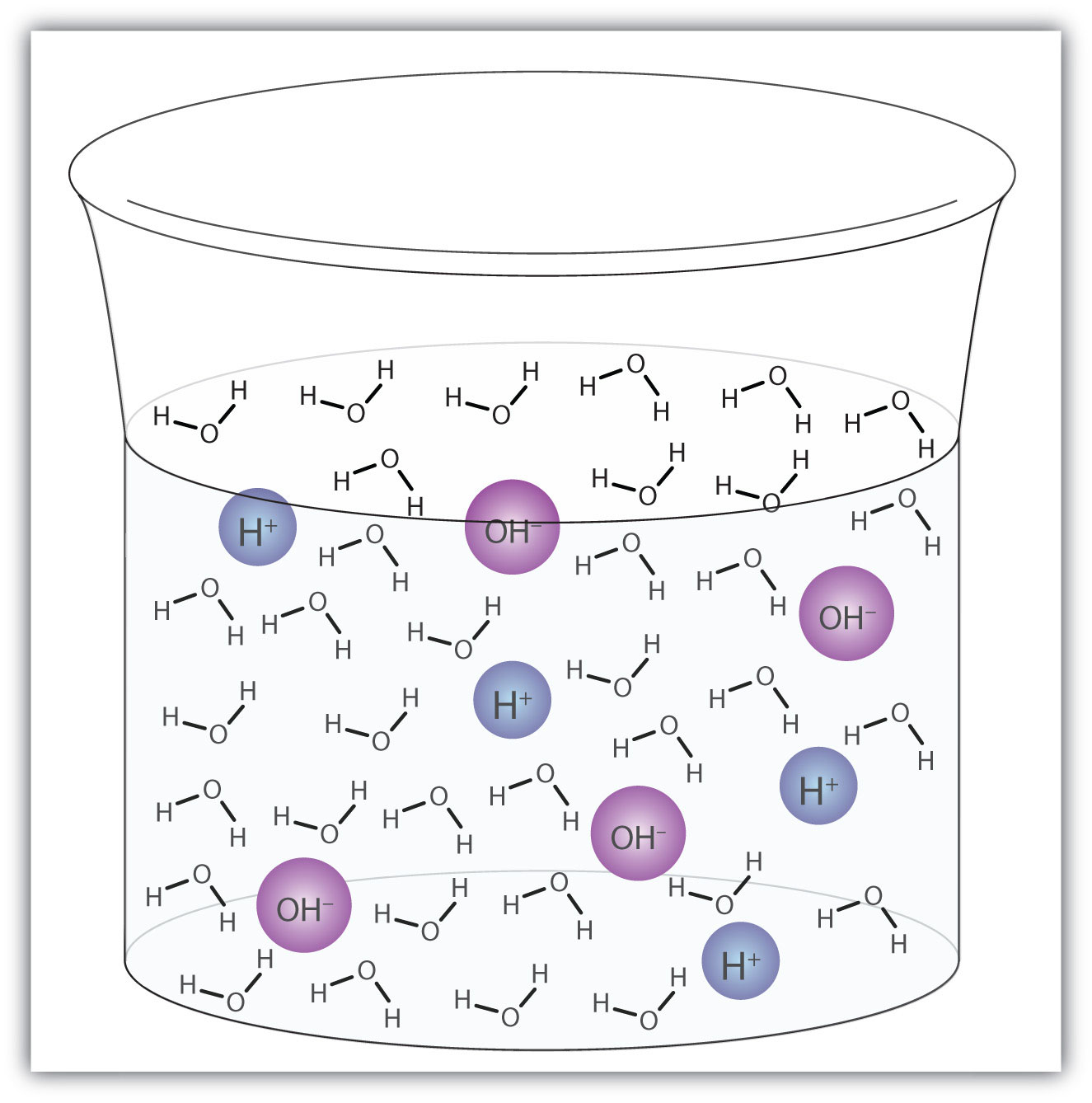This is “Water: Both an Acid and a Base”, section 10.3 from the book Introduction to Chemistry: General, Organic, and Biological (v. 1.0). For details on it (including licensing), click here.

Has this book helped you? Consider passing it on:
Creative Commons supports free culture from music to education. Their licenses helped make this book available to you.
DonorsChoose.org helps people like you help teachers fund their classroom projects, from art supplies to books to calculators.

## 10.3 Water: Both an Acid and a Base

### Learning Objective

1. Write chemical equations for water acting as an acid and as a base.

Water (H2O) is an interesting compound in many respects. Here, we will consider its ability to behave as an acid or a base.

In some circumstances, a water molecule will accept a proton and thus act as a Brønsted-Lowry base. We saw an example in the dissolving of HCl in H2O:

HCl + H2O(ℓ) → H3O+(aq) + Cl(aq)

In other circumstances, a water molecule can donate a proton and thus act as a Brønsted-Lowry acid. For example, in the presence of the amide ion (see Example 4 in Section 10.2 "Brønsted-Lowry Definition of Acids and Bases"), a water molecule donates a proton, making ammonia as a product:

H2O(ℓ) + NH2(aq) → OH(aq) + NH3(aq)

In this case, NH2 is a Brønsted-Lowry base (the proton acceptor).

So, depending on the circumstances, H2O can act as either a Brønsted-Lowry acid or a Brønsted-Lowry base. Water is not the only substance that can react as an acid in some cases or a base in others, but it is certainly the most common example—and the most important one. A substance that can either donate or accept a proton, depending on the circumstances, is called an amphiproticA substance that can either donate or accept a proton, depending on the circumstances. compound.

A water molecule can act as an acid or a base even in a sample of pure water. About 6 in every 100 million (6 in 108) water molecules undergo the following reaction:

H2O(ℓ) + H2O(ℓ) → H3O+(aq) + OH(aq)

This process is called the autoionization of waterThe process by which water ionizes into hydronium ions and hydroxide ions as it acts as an acid and a base. (Figure 10.2 "Autoionization") and occurs in every sample of water, whether it is pure or part of a solution. Autoionization occurs to some extent in any amphiprotic liquid. (For comparison, liquid ammonia undergoes autoionization as well, but only about 1 molecule in a million billion (1 in 1015) reacts with another ammonia molecule.)

Figure 10.2 AutoionizationA small fraction of water molecules—approximately 6 in 100 million—ionize spontaneously into hydronium ions and hydroxide ions. This picture necessarily overrepresents the amount of autoionization that really occurs in pure water.

### Example 5

Identify water as either a Brønsted-Lowry acid or a Brønsted-Lowry base.

1. H2O(ℓ) + NO2(aq) → HNO2(aq) + OH(aq)
2. HC2H3O2(aq) + H2O(ℓ) → H3O+(aq) + C2H3O2(aq)

Solution

1. In this reaction, the water molecule donates a proton to the NO2 ion, making OH(aq). As the proton donor, H2O acts as a Brønsted-Lowry acid.
2. In this reaction, the water molecule accepts a proton from HC2H3O2, becoming H3O+(aq). As the proton acceptor, H2O is a Brønsted-Lowry base.

### Skill-Building Exercise

Identify water as either a Brønsted-Lowry acid or a Brønsted-Lowry base.

1. HCOOH(aq) + H2O(ℓ) → H3O+(aq) + HCOO(aq)

2. H2O(ℓ) + PO43−(aq) → OH(aq) + HPO42−(aq)

### Concept Review Exercises

1. Explain how water can act as an acid.

2. Explain how water can act as a base.

1. Under the right conditions, H2O can donate a proton, making it a Brønsted-Lowry acid.

2. Under the right conditions, H2O can accept a proton, making it a Brønsted-Lowry base.

### Key Takeaway

• Water molecules can act as both an acid and a base, depending on the conditions.

### Exercises

1. Is H2O(ℓ) acting as an acid or a base?

H2O(ℓ) + NH4+(aq) → H3O+(aq) + NH3(aq)
2. Is H2O(ℓ) acting as an acid or a base?

CH3(aq) + H2O(ℓ) → CH4(aq) + OH(aq)
3. In the aqueous solutions of some salts, one of the ions from the salt can react with water molecules. In some C2H3O2 solutions, the following reaction can occur:

C2H3O2(aq) + H2O(ℓ) → HC2H3O2(aq) + OH(aq)

Is H2O acting as an acid or a base in this reaction?

4. In the aqueous solutions of some salts, one of the ions from the salt can react with water molecules. In some NH4+ solutions, the following reaction can occur:

NH4+(aq) + H2O → NH3(aq) + H3O+(aq)

Is H2O acting as an acid or a base in this reaction?

5. Aluminum hydroxide [Al(OH)3] is amphoteric; it reacts with both acids and bases. Propose the chemical equations for the reactions of Al(OH)3 with H+ and with OH.

6. Based on the information in this section, does ammonia (NH3) autoionize more or less than water? Write the chemical equation for the autoionization of ammonia.